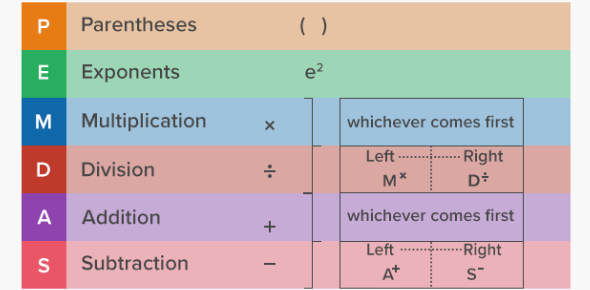# Order Of Operations Exam! MCQ Quiz

22 Questions | Total Attempts: 245SettingsIn mathematics, the order of operations refers to a cluster of rules that reflect an agreement about which methodology to execute to assess a given mathematical expression. This quiz will test your mathematical skills in solving algebraic equations. Read the questions carefully and answer.

• 1.
92 × 1 − (92 × 9) × 4
• A.

-2835

• B.

-2245

• C.

2835

• D.

2245

• 2.
(3 − 3) ÷ 7
• A.

4

• B.

0

• C.

5

• D.

2

• 3.
(92 + 62) − 2
• A.

115

• B.

100

• C.

85

• D.

110

• 4.
(1 + 62 + 9)
• A.

89

• B.

12

• C.

25

• D.

46

• 5.
(7 + 8) + 5 − 8
• A.

12

• B.

24

• C.

36

• D.

-15

• 6.
(2 × 5) + 5
• A.

12

• B.

15

• C.

14

• D.

13

• 7.
(8 + 8 + 8)
• A.

17

• B.

-52

• C.

13

• D.

24

• 8.
(12 + 3) × 8
• A.

32

• B.

27

• C.

12

• D.

9

• 9.
2 × 2 + (7 × 7) + 7
• A.

20

• B.

40

• C.

60

• D.

15

• 10.
3 + 82 ÷ (8 − 4) ÷ 2
• A.

11

• B.

13

• C.

12

• D.

17

• 11.
(72 − 3 × 9)
• A.

22

• B.

14

• C.

18

• D.

16

• 12.
(22 − 22) × 12 ÷ 5
• A.

2

• B.

-7

• C.

0

• D.

15

• 13.
9 × (9 + 3) ÷ 4
• A.

22

• B.

19

• C.

21

• D.

27

• 14.
(52 × 4) ÷ 2
• A.

12

• B.

50

• C.

17

• D.

65

• 15.
(5 + 8 + 7 − 2)
• A.

14

• B.

16

• C.

17

• D.

18

• 16.
(5 ÷ 1) − 8 + 5
• A.

2

• B.

5

• C.

7

• D.

3

• 17.
(62 ÷ 62) + 5
• A.

1

• B.

4

• C.

25

• D.

6

• 18.
(8 ÷ 22 − 1)
• A.

1

• B.

0

• C.

2

• D.

3

• 19.
(3 − 4 − 5)
• A.

-6

• B.

-25

• C.

12

• D.

-12

• 20.
(3 × 32 + 7)
• A.

34

• B.

10

• C.

15

• D.

38

• 21.
(5 − 9) + (3 + 4) × 7
• A.

9

• B.

15

• C.

22

• D.

45

• 22.
(92 − 5 + 1)
• A.

25

• B.

43.5

• C.

18

• D.

77

Related TopicsBack to top